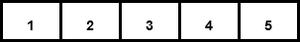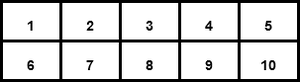Open in App
Not now

# Basics of NumPy Arrays

• Difficulty Level : Easy
• Last Updated : 26 Apr, 2022

NumPy stands for Numerical Python. It is a Python library used for working with an array. In Python, we use the list for purpose of the array but it’s slow to process. NumPy array is a powerful N-dimensional array object and its use in linear algebra, Fourier transform, and random number capabilities. It provides an array object much faster than traditional Python lists.

#### Types of Array:

1. One Dimensional Array
2. Multi-Dimensional Array

#### One Dimensional Array:

A one-dimensional array is a type of linear array.One Dimensional Array

Example:

## Python3

 `# importing numpy module``import` `numpy as np` `# creating list``list` `=` `[``1``, ``2``, ``3``, ``4``]` `# creating numpy array``sample_array ``=` `np.array(list1)` `print``(``"List in python : "``, ``list``)` `print``(``"Numpy Array in python :"``,``      ``sample_array)`

Output:

```List in python :  [1, 2, 3, 4]
Numpy Array in python :  [1 2 3 4]```

Check data type for list and array:

## Python3

 `print``(``type``(list_1))` `print``(``type``(sample_array))`

Output:

```<class 'list'>
<class 'numpy.ndarray'>```

#### Multi-Dimensional Array:

Data in multidimensional arrays are stored in tabular form.Two Dimensional Array

Example:

## Python3

 `# importing numpy module``import` `numpy as np` `# creating list``list_1 ``=` `[``1``, ``2``, ``3``, ``4``]``list_2 ``=` `[``5``, ``6``, ``7``, ``8``]``list_3 ``=` `[``9``, ``10``, ``11``, ``12``]` `# creating numpy array``sample_array ``=` `np.array([list_1,``                         ``list_2,``                         ``list_3])` `print``(``"Numpy multi dimensional array in python\n"``,``      ``sample_array)`

Output:

```Numpy multi dimensional array in python
[[ 1  2  3  4]
[ 5  6  7  8]
[ 9 10 11 12]]```

Note: use [ ] operators inside numpy.array() for multi-dimensional

#### Anatomy of an array :

1. Axis: The Axis of an array describes the order of the indexing into the array.

Axis 0 = one dimensional

Axis 1 = Two dimensional

Axis 2 = Three dimensional

2. Shape: The number of elements along with each axis. It is from a tuple.

Example:

## Python3

 `# importing numpy module``import` `numpy as np` `# creating list``list_1 ``=` `[``1``, ``2``, ``3``, ``4``]``list_2 ``=` `[``5``, ``6``, ``7``, ``8``]``list_3 ``=` `[``9``, ``10``, ``11``, ``12``]` `# creating numpy array``sample_array ``=` `np.array([list_1,``                         ``list_2,``                         ``list_3])` `print``(``"Numpy array :"``)``print``(sample_array)` `# print shape of the array``print``(``"Shape of the array :"``,``      ``sample_array.shape)`

Output:

```Numpy array :
[[ 1  2  3  4]
[ 5  6  7  8]
[ 9 10 11 12]]
Shape of the array :  (3, 4)```

Example:

## Python3

 `import` `numpy as np` `sample_array ``=` `np.array([[``0``, ``4``, ``2``],``                       ``[``3``, ``4``, ``5``],``                       ``[``23``, ``4``, ``5``],``                       ``[``2``, ``34``, ``5``],``                       ``[``5``, ``6``, ``7``]])` `print``(``"shape of the array :"``,``      ``sample_array.shape)`

Output:

`shape of the array :  (5, 3)`

3. Rank: The rank of an array is simply the number of axes (or dimensions) it has.

The one-dimensional array has rank 1.Rank 1

The two-dimensional array has rank 2.Rank 2

4. Data type objects (dtype): Data type objects (dtype) is an instance of numpy.dtype class. It describes how the bytes in the fixed-size block of memory corresponding to an array item should be interpreted.

Example:

## Python3

 `# Import module``import` `numpy as np` `# Creating the array``sample_array_1 ``=` `np.array([[``0``, ``4``, ``2``]])` `sample_array_2 ``=` `np.array([``0.2``, ``0.4``, ``2.4``])` `# display data type``print``(``"Data type of the array 1 :"``,``      ``sample_array_1.dtype)` `print``(``"Data type of array 2 :"``,``      ``sample_array_2.dtype)`

Output:

```Data type of the array 1 :  int32
Data type of array 2 :  float64```

Some different way of creating Numpy Array :

1. numpy.array(): The Numpy array object in Numpy is called ndarray. We can create ndarray using numpy.array() function.

Syntax: numpy.array(parameter)

Example:

## Python3

 `# import module``import` `numpy as np` `#creating a array` `arr ``=` `np.array([``3``,``4``,``5``,``5``])` `print``(``"Array :"``,arr)`

Output:

`Array : [3 4 5 5]`

2. numpy.fromiter(): The fromiter() function create a new one-dimensional array from an iterable object.

Syntax: numpy.fromiter(iterable, dtype, count=-1)

Example 1:

## Python3

 `#Import numpy module``import` `numpy as np` `# iterable``iterable ``=` `(a``*``a ``for` `a ``in` `range``(``8``))` `arr ``=` `np.fromiter(iterable, ``float``)` `print``(``"fromiter() array :"``,arr)`

Output:

fromiter() array :  [ 0.  1.  4.  9. 16. 25. 36. 49.]

Example 2:

## Python3

 `import` `numpy as np` `var ``=` `"Geekforgeeks"` `arr ``=` `np.fromiter(var, dtype ``=` `'U2'``)` `print``(``"fromiter() array :"``,``      ``arr)`

Output:

fromiter() array :  [‘G’ ‘e’ ‘e’ ‘k’ ‘f’ ‘o’ ‘r’ ‘g’ ‘e’ ‘e’ ‘k’ ‘s’]

3. numpy.arange(): This is an inbuilt NumPy function that returns evenly spaced values within a given interval.

Syntax: numpy.arange([start, ]stop, [step, ]dtype=None)

Example:

## Python3

 `import` `numpy as np` `np.arange(``1``, ``20` `, ``2``,``          ``dtype ``=` `np.float32)`

Output:

array([ 1.,  3.,  5.,  7.,  9., 11., 13., 15., 17., 19.], dtype=float32)

4. numpy.linspace(): This function returns evenly spaced numbers over a specified between two limits.

Syntax: numpy.linspace(start, stop, num=50, endpoint=True, retstep=False, dtype=None, axis=0)

Example 1:

## Python3

 `import` `numpy as np` `np.linspace(``3.5``, ``10``, ``3``)`

Output:

`array([ 3.5 ,  6.75, 10.  ])`

Example 2:

## Python3

 `import` `numpy as np` `np.linspace(``3.5``, ``10``, ``3``,``            ``dtype ``=` `np.int32)`

Output:

`array([ 3,  6, 10])`

5. numpy.empty(): This function create a new array of given shape and type, without initializing value.

Syntax: numpy.empty(shape, dtype=float, order=’C’)

Example:

## Python3

 `import` `numpy as np` `np.empty([``4``, ``3``],``         ``dtype ``=` `np.int32,``         ``order ``=` `'f'``)`

Output:

```array([[ 1,  5,  9],
[ 2,  6, 10],
[ 3,  7, 11],
[ 4,  8, 12]])```

6. numpy.ones(): This function is used to get a new array of given shape and type, filled with ones(1).

Syntax: numpy.ones(shape, dtype=None, order=’C’)

Example:

## Python3

 `import` `numpy as np` `np.ones([``4``, ``3``],``        ``dtype ``=` `np.int32,``        ``order ``=` `'f'``)`

Output:

```array([[1, 1, 1],
[1, 1, 1],
[1, 1, 1],
[1, 1, 1]])```

7. numpy.zeros(): This function is used to get a new array of given shape and type, filled with zeros(0).

Syntax: numpy.ones(shape, dtype=None)

Example:

## Python3

 `import` `numpy as np``np.zeros([``4``, ``3``],``         ``dtype ``=` `np.int32,``         ``order ``=` `'f'``)`

Output:

```array([[0, 0, 0],
[0, 0, 0],
[0, 0, 0],
[0, 0, 0]])```

My Personal Notes arrow_drop_up